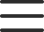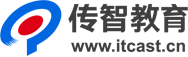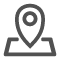# java 实现接口

6.2 实现接口

1 类实现接口

再如，Animal 的Dog子类实现Eatable和Sleepable接口。

classDog extends Aninal implements Eatable,Sleepable

2.重写接口中的方法

如果一个非抽象类实现了某个接口，那么这个类必须重写这个接口中的所有方法。需要注意的是，由于接口中的方法定是public abstract 方法，所以类在重写接口方法时不仅要去掉abstract 修饰符、给出方法体，而且方法的访问权限一定要明显地用public来修饰(否则就降低了访问权限，这是不允许的).实现接口的非抽象类实现了该接口中的方法，即给出了方法的具体行为功能。用户也可以自定义接口，一个Java源文件可以由类和接口组成。

下面的例子1中包含China类、Japan类和Compuable接口，而且China类和Japan类都实现了Computable接口。

Computable.java

• public interface Computable{

•         int MAX=46;

•         int f(int x);

• }

China.java

• class China implements Computable{ //China类实现Computable接口

•         int number;

•         public int f(int x){       //不要忘记publie关键宇

•                 int sum=0;

•                 for(int i=0;i<=x;i++){

•                         sum=sum+i;

•                 }

•                 return  sum;

•         }

• }

Japan.java

• class Japan implements Computable { //Japan 类实现Computable接口

•         int number;

•         public int f(int x){

•                 return MAX+x; //直接使用接口中的常量

•         }

• }

Example6_1.java

• public class Example6_1{

•         public static void main(String args[]){

•                 China zhang;

•                 Japan henlu;

•                 zhang=new China();

•                 henlu=new Japan();

•                 zhang.number=32+Computable .MAX; //用接口名访问接口的常量

•                 henlu.number=14+Computable.MAX;

•                 System.out.println("zhang的学号"+zhang.number+",zhang 求和结果"+zhang.f(100));

•                 System.out.println("henlu的学号"+henlu.number+",henlu 求和结果"+henlu.f(100));

•         }

• }

• interface Computable {

•     final int MAX = 100;

•     vo1d speak(String s);

•     int f(int x);

•     float g(float x,float y);

• }

• abstract classA implements Computable{

•     public int f(int x){

•         int sum 0:

•         for(int i=1;i<=x;i++) {

•             sum=sum+i;

•         }

•     return sum;

•     }

• }

4.接口的细节说明

程序可以用接口名方问接口中的常量，但是如果个类实现了接口，那么该类可以直接在类体中使用该接口中的常量。

如果父类实现了某个接口，那么子类也就自然实现了该接口，子类不必再显式地使用关键字implements声明实现这个接口，

接口也可以被继承，即可以通过关键字extends声明个接口是另个接口的 子接口。由于接口中的方法和常量都是public的，子接口将继承父接口中的全部方法和常量。

• 注: Java提供的接口都在相应的包中，通过import语向不仅可以引入包中的类，也可以引入包中的接口，例如:

•     importJava.io.*;

• 不仅引入了java.io包中的类，同时也引入了该包中的接口。
•